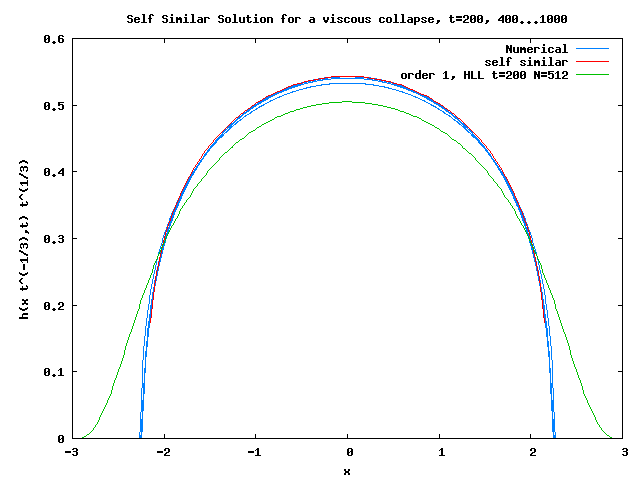# Collapse of a rectangular viscous column,

or collapse of a viscous fluid (double viscous dam break)

From the paper by Huppert 82 “The propagation of two-dimensional and axisymmetric viscous gravity currents over a rigid horizontal surface”

We solve shallow water in 1D. \displaystyle \left\{\begin{array}{l} \partial_t h+\partial_x Q=0\\ \partial_t Q+ \partial_x \left[\dfrac{Q^2}{h}+g\dfrac{h^2}{2}\right] = - C_f Q h^{-2} \end{array}\right. where the friction is laminar C_f=3 and with at t=0, a given heap h(|x|<4,t=0)=.25 so that \int_{-\infty}^{\infty} h(x,0)dx =2.

as the flow is very viscous, the convective term is small, and there only is a balance between pressure gradient and viscosity so that the flux is (lubrication equation): \displaystyle Q \simeq - \frac{g h^3}{3\nu} \partial_x h in the mass conservation, this gives \displaystyle \frac{\partial}{\partial t} h - (\frac{g}{3\nu})\frac{\partial}{\partial x} (h^3 \frac{\partial}{\partial x} h)\simeq 0 as this equation has a self similar solution, we compare to it.

#include "grid/cartesian1D.h"
#include "saint-venant.h"

// Huppert 82 "The propagation of two-dimensional and axisymmetric
// viscous gravity currents over a rigid horizontal surface"

// declare parameters
double Cf;
double tmax;

u.n[left]   = - radiation(0);
u.n[right]  = + radiation(0);

start by a rectangular column of surface 2

event init (i = 0)
{
foreach(){
zb[] =  0;
h[] = (0.5*(fabs(x)<2));}
}

split for friction \displaystyle \frac{\partial u}{\partial t} = -C_f \frac{u}{h^2} \text { so that a semi implicit discretization gives } \frac{ u^{n+1} - u^n }{\Delta t} = -C_f \frac{u^{u+1}}{h^{n2}} we impose u^{n+1}=0 when h^n very small (parameter dry).

event friction (i++) {
// Poiseuille friction (implicit scheme)
foreach()
{  	 double ff = h[] < dry ? HUGE : (1. + Cf*dt/h[]/h[]);
u.x[] /= ff;
}
}

saving some profiles in files eta0 eta1 eta2… extracted from the output (obsolete, comes from version 1 of Basilisk)

event outputfile (t <= tmax;t+=200) {
static int nf = 0;
fprintf (stderr,"#file: eta-%d\n", nf++);
foreach()
fprintf (stderr,"%g %g %g \n", x, h[], t );
}

saving in self similar variable

event field (t <= tmax;t+=200) {
foreach()
printf ("p %g %g %g %g \n", x/pow(t+1e-9,.2), h[]*pow(t,.2), u.x[], zb[]);
printf ("p\n");
}

end of subroutines

position of domain X0, length L0, no dimension G=1 run with 2048 points (less is not enough)

int main() {
X0 = -15./2;
Y0 = -15./2;
L0 = 30./2;
G = 1.;
Cf = 3.;
N = 2048*4;
tmax=1000;

run();
}

# Results

To compile and run

qcc -g -O2 -DTRASH=1 -Wall -o viscous_collapse viscous_collapse.c -lm
./viscous_collapse > viscous_collapse.out 2> viscous_collapse.out2 

using make

make viscous_collapse.tst
make viscous_collapse/plots
make viscous_collapse.c.html

source c2html.sh viscous_collapse

once it is finished, two png files are generated (obsolete, comes from version 1 of Basilisk).

  awk '{ if ($1 == "#file:") file =$2; else print $0 > file; }' < log plot [][:.3]'eta-0' not w l lc -1,'eta-1'not w l lc -1,\ 'eta-2'not w l lc -1,'eta-3'not w l lc -1,'eta-4'not w l lc -1,'eta-5'not w l lc -1  set xlabel 'x' set ylabel 'h(x,t)' set arrow from 0,.47 to 0,0.08 set label "t" at 0.2,0.1 set arrow from 3,.05 to 7,0.05 set label "t" at 8,0.06 p [-10:10][:1] 'log' w l lc -1 notcollapse (script) gives an example of collapse Self similar solution \eta=xt^{-1/5}, with b=1.13286 and h_0=1.04922, front position x_f=b^{1/5}: \displaystyle h(\eta) = h_0 (1-(\frac{\eta}{b})^2)^{1/3}  reset set title 'Self Similar Solution for a viscous collapse, t=200, 400...1000' set xlabel 'x' set ylabel 'h(x t^{-1/5},t) t^{1/5}' b=1.13286 h(x) =1.04922* ((1-x*x/b/b))**(1/3.) bed(x) = 0 set key top right plot [-2:2][0:2] \ '< grep ^p out' u 2:3 w l lc 3 t 'Numerical', \ h(x) lw 1 lc 1 lt 1 t 'self similar'collapse (script) # Comparison with an simplier code collapse over the selfsimilar solution for Cf=.125 Self similar solution, b=2.18812 and h_0=0.543217 \displaystyle h(x) = h_0 (1-(\frac{x}{b})^2)^{1/3} 'viscous_collapse.ref' u ($1/t5):(\$2*t5) t'order 1, HLL t=200 N=512' w l  lc 2Huppert’s self similar solution

Version 1: Sydney Juillet 13, OK v2